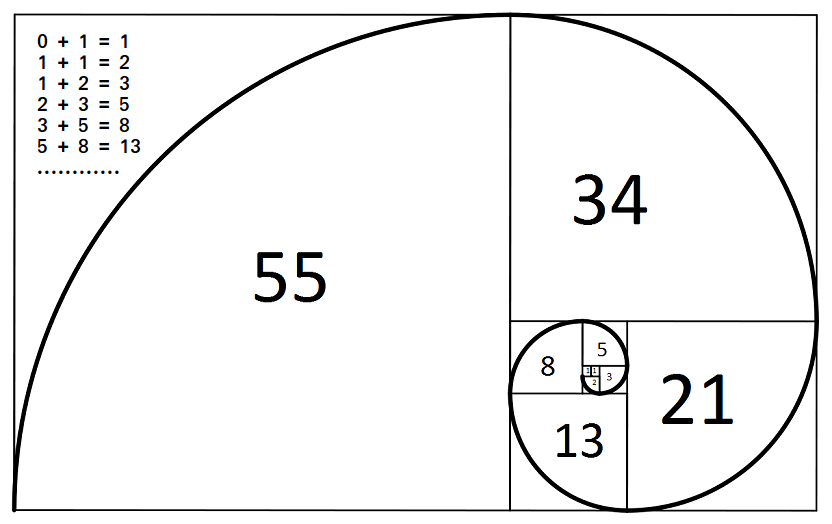# Program to print first n Fibonacci Numbers | Set 1

Following is simple program to print first n Fibonacci numbers.Examples :

```Input : n = 3
Output : 1 1 2

Input : n = 7
Output : 1 1 2 3 5 8 13
```

## C++

 `// C++ program to print first n Fibonacci numbers  ` `#include ` `using` `namespace` `std;  ` ` `  `// Function to print first n Fibonacci Numbers  ` `void` `printFibonacciNumbers(``int` `n)  ` `{  ` `    ``int` `f1 = 0, f2 = 1, i;  ` ` `  `    ``if` `(n < 1)  ` `        ``return``;  ` ` `  `    ``for` `(i = 1; i <= n; i++)  ` `    ``{  ` `        ``cout<

## C

 `// C program to print first n Fibonacci numbers ` `#include ` ` `  `// Function to print first n Fibonacci Numbers ` `void` `printFibonacciNumbers(``int` `n) ` `{ ` `    ``int` `f1 = 0, f2 = 1, i; ` ` `  `    ``if` `(n < 1) ` `        ``return``; ` ` `  `    ``for` `(i = 1; i <= n; i++) ` `    ``{ ` `        ``printf``(``"%d "``, f2); ` `        ``int` `next = f1 + f2; ` `        ``f1 = f2; ` `        ``f2 = next; ` `    ``} ` `} ` ` `  `// Driver Code ` `int` `main() ` `{ ` `    ``printFibonacciNumbers(7); ` `    ``return` `0; ` `} `

## Java

 `// Java program to print first n Fibonacci Numbers ` ` `  `class` `Test ` `{ ` `    ``// Method to print first n Fibonacci Numbers ` `    ``static` `void` `printFibonacciNumbers(``int` `n) ` `    ``{ ` `        ``int` `f1 = ``0``, f2 = ``1``, i; ` `     `  `        ``if` `(n < ``1``) ` `            ``return``; ` `     `  `        ``for` `(i = ``1``; i <= n; i++) ` `        ``{ ` `            ``System.out.print(f2+``" "``); ` `            ``int` `next = f1 + f2; ` `            ``f1 = f2; ` `            ``f2 = next; ` `        ``} ` `    ``}  ` `     `  `    ``// Driver Code ` `    ``public` `static` `void` `main(String[] args)  ` `    ``{ ` `        ``printFibonacciNumbers(``7``); ` `    ``} ` `} `

## Python3

 `# Python program to print first n  ` `# Fibonacci numbers ` ` `  `# Function to print first n  ` `# Fibonacci Numbers ` `def` `printFibonacciNumbers(n): ` `     `  `    ``f1 ``=` `0` `    ``f2 ``=` `1` `    ``if` `(n < ``1``): ` `        ``return` `    ``for` `x ``in` `range``(``0``, n): ` `        ``print``(f2, end ``=` `" "``) ` `        ``next` `=` `f1 ``+` `f2 ` `        ``f1 ``=` `f2 ` `        ``f2 ``=` `next` `         `  `# Driven code ` `printFibonacciNumbers(``7``) ` ` `  `# This code is contributed by Danish Raza `

## C#

 `// C# program to print first n Fibonacci Numbers ` `using` `System; ` ` `  `class` `Test ` `{ ` `    ``// Method to print first n Fibonacci Numbers ` `    ``static` `void` `printFibonacciNumbers(``int` `n) ` `    ``{ ` `        ``int` `f1 = 0, f2 = 1, i; ` `     `  `        ``if` `(n < 1) ` `            ``return``; ` `     `  `        ``for` `(i = 1; i <= n; i++) ` `        ``{ ` `            ``Console.Write(f2 + ``" "``); ` `            ``int` `next = f1 + f2; ` `            ``f1 = f2; ` `            ``f2 = next; ` `        ``} ` `    ``}  ` `     `  `    ``// Driver Code ` `    ``public` `static` `void` `Main()  ` `    ``{ ` `        ``printFibonacciNumbers(7); ` `    ``} ` `} ` ` `  `// This code is contributed by nitin mittal. `

## PHP

 ` `

Output:

`1 1 2 3 5 8 13`

Time Complexity : O(n)

If you like GeeksforGeeks and would like to contribute, you can also write an article using contribute.geekforgeeks.org or mail your article to contribute@geeksforgeeks.org. See your article appearing on the GeeksforGeeks main page and help other Geeks.